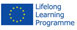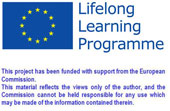This project has been funded with support from the European Commission.
This material reflects the views only of the author, and the Commission cannot be held responsible for any use which may be made of the information contained therein

Also available in: bg es it lv pl ro sk en fb# Experiences

Homepage > Experiences > Teachers Experiences

TEACHERS EXPERIENCES FORM

Title of the Experience
Exponential equation
Name of the teacher
Country where it took place
Slovakia
School typology
High Secondary School
Thematic Area
Maths
Experience typology
Teaching in class
Type of contact
Direct
Description of the Experience
The aim of this theme unit is similar to teaching thematic unit on exponential functions. The main goal consisted of linking students' knowledge about the powers of resolving issues in technical subjects leading to an exponential equation (calculation of values in relations, formulas, electronics and mechanical engineering). At the same time, pupils were presented how to solve various mathematical problems not only developing their mathematical and logic skills but also supporting memory processes and developing critical thinking. It promotes the ability to sort information and then use those which are necessary for solving the problem.

The thematic unit of Exponential equation follows directly after the unit of Exponential functions.
The students are introduced to the equations issue with a help of the construction of complex graphs of exponential functions (without a PC) when positioning accuracy of the graph of the function is increasing by finding important points of the function, for example, intersection points of the axes of the coordinate system.
Exponential equations were solved increasingly from the simpler, the solution of which were sufficient knowledge about defining powers, to more complex, for which it was necessary to use the formula of compassion, share, augmented with squares and follow the same basic equation, the solution of which it was necessary to use a substitution leading to the solution linear or quadratic equation. The challenges of this thematic unit were completed by drawing graphs without using a PC (in mathematics) and using the PC as a way to verify the correctness of task solution for home project.
Students could have used the webpage in preparation for the class. I started preparing the documents of the website according to their wish so that the worksheets were at the beginning of the core elements of the curriculum and part of the task was solved.

Task solving of the exponential equation is the curriculum that is taught after getting familiar with the curriculum of the exponential function. For a successful understanding it is necessary to cope very well with the curriculum of the first year which consists of the square and solving linear and quadratic equations.
Challenges to the successful implementation of teaching this subject matter were similar to the previous curriculum topic:
1. Lack of knowledge about the activities of the squares
2. Insecure knowledge of the curriculum of equations and inequalities of various kinds
However,I can note that the students have mastered the curriculum at a higher level than the subject matter of the exponential equation.
They had problems mostly with squares with a negative exponent (after school in the extracurricular time we studied with the students solving the tasks in which they investigated the relationship between squares with integer exponent) and in solving equations using substitution when they forgot to implement the re-substitution (also in extracurricular time I have with those who have expressed interest addressed the problems for which it is appropriate but not necessary to use a substitution).

Recommended web: www.kolbi.wbl.sk.
Unfortunately I still have to say that pupils used the website as well as the opportunity to “remove their weaknesses in their knowledge” in small scale.
Also this time the most effective solution to problem solving was using the worksheets and that mostly individually for additional points to their evaluation.
After my previous experience I allowed students to use their “cheating notes- formulas” which was evaluated positively from their point of view.